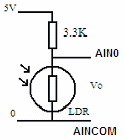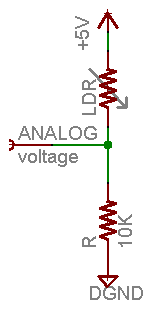# Measure light intensity

Hi,

I'm posting this message, but i'm not completely sure if it's the best section. Anyway, I have a mathematical problem related to this LDR equation:

What I don't understand is the reworking of the equation and how the result becomes:

Lux = (2500/Vo - 500)/3.3

Thanks,

Not surprising as its wrong!

Algebra:
Vout / Vin = R / (R + 3.3)
Vout / 5 = R / (R + 3.3) = (500/lux) / (500/lux + 3.3) = 500 / (500 + 3.3 lux)

therefore

500 + 3.3 lux = 500 (5 / Vout)

3.3 lux = 500 (5 / Vout - 1)

lux = 500 (5 / Vout - 1) / 3.3

you mean these steps:

1. RL= 500/lux
2. Vo = 5*RL / (RL+3.3)

fill in 1 into 2

1. Vo = 5*500/Lux / (500/lux +3.3)
2. Vo = 2500/Lux / (500/lux +3.3)

rewrite A/B/C == A/(B*C)

1. Vo = 2500/ (500 +3.3*lux)

divide by 2500

1. Vo/2500 = 1/(500 +3.3*lux)

invert

1. 2500/Vo = 500 + 3.3*lux

solve for lux
8] 3.3*lux = 2500/Vo - 500
9) lux = (2500/Vo - 500)/3.3

So I think the formula in the PDF is right too as

lux = 500 (5 / Vout - 1) / 3.3
<>
lux = (2500/Vout - 500)/3.3

Thanks Rob,

Now I understand it

robtillaart:
So I think the formula in the PDF is right too as

lux = 500 (5 / Vout - 1) / 3.3
<>
lux = (2500/Vout - 500)/3.3

I have a question sir, is it accurate ?
I keep getting High Lux in dark while I get low lux in bright ? is it correct? or there's something wrong with my equation ? by the way I used the equation you stated.

Possibly you have coded it wrong or you're using different components or you have components wired wrong.

If you provide more information then it may be possible to help.

Steve

silentzer14:
I keep getting High Lux in dark while I get low lux in bright ?

The diagram linked in the opening post shows the LDR as the bottom resistor in the divider:Other sites eg adafruit show it as the top:The equation in this thread would be derived for the first case; how is yours wired?

I have a question sir, is it accurate ?

No. LDRs are highly nonlinear. They are useful to tell whether, for example, the illumination is very bright, bright, dim or dark.

If you want to measure light intensity with any accuracy at all, use a photodiode and a suitable amplifier.

You could try a MAX44009 instead of a LDR. It returns the number as LUX.

The TSL2591 is another inexpensive lux meter. Almost certainly much more suitable for the job at hand.